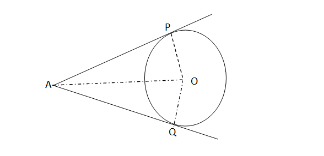# If two tangents are drawn to a circle from an external point,show`
Question:

If two tangents are drawn to a circle from an external point,show that they subtend equal angles at the centre.

Solution:Given : A circle with centre O and a point A outside it. Also, AP and AQ are the two tangents to the circle.

:To prove: $\angle A O P=\angle A O Q$.

Proof : In $\Delta A O P$ and $\Delta A O Q$, we have :

$A P=A Q \quad$ [tangents from an external point are equal]

$O P=O Q \quad$ [radii of the same circle]

$O A=O A \quad[$ common side $]$

$\therefore \Delta A O P \cong \Delta A O Q \quad$ [by SSS - congruence]

Hence, $\angle A O P=\angle A O Q$ (c. p. c. $\mathrm{t}$ ).